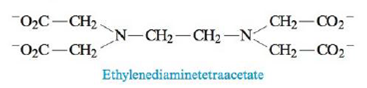# Calculate the concentration of Pb 2+ in each of the following. a. a saturated solution of Pb(OH) 2 , K sp = 1.2 × 10 −15 b. a saturated solution of Pb(OH) 2 buffered at pH = 13.00 c. Ethylenediaminetetraacetate (EDTA 4− ) is used as a complexing agent in chemical analysis and has the following structure: Solutions of EDTA 4− are used to treat heavy metal poisoning by removing the heavy metal in the form of a soluble complex ion. The reaction of EDTA 4− with Pb 2+ is Pb 2 + ( a q ) + E D T A 4 − ( a q ) ⇌ P b E D T A 2 − ( a q ) K = 1.1 × 10 18 Consider a solution with 0.010 mole of Pb(NO 3 ) 2 added to 1.0 L of an aqueous solution buffered at pH = 13.00 and containing 0.050 M Na 4 .EDTA. Does Pb(OH) 2 precipitate from this solution?### Chemistry: An Atoms First Approach

2nd Edition
Steven S. Zumdahl + 1 other
Publisher: Cengage Learning
ISBN: 9781305079243

#### Solutions

Chapter
Section### Chemistry: An Atoms First Approach

2nd Edition
Steven S. Zumdahl + 1 other
Publisher: Cengage Learning
ISBN: 9781305079243
Chapter 15, Problem 87AE
Textbook Problem
1 views

## Calculate the concentration of Pb2+ in each of the following.a. a saturated solution of Pb(OH)2, Ksp = 1.2 × 10−15b. a saturated solution of Pb(OH)2 buffered at pH = 13.00c. Ethylenediaminetetraacetate (EDTA4−) is used as a complexing agent in chemical analysis and has the following structure:Solutions of EDTA4− are used to treat heavy metal poisoning by removing the heavy metal in the form of a soluble complex ion. The reaction of EDTA4− with Pb2+ is Pb 2 + ( a q )   +   E D T A 4 −   ( a q )   ⇌   P b E D T A 2 − ( a q )   K   =   1.1   ×   10 18 Consider a solution with 0.010 mole of Pb(NO3)2 added to 1.0 L of an aqueous solution buffered at pH = 13.00 and containing 0.050 M Na4.EDTA. Does Pb(OH)2 precipitate from this solution?

(a)

Interpretation Introduction

Interpretation: The concentration of Pb2+ is to be calculated in each of the given cases.

Concept introduction: Solubility is defined as the maximum amount of solute that can dissolve at a certain amount of solvent at certain temperature. The solubility product, Ksp is the equilibrium constant that is applied when salt partially dissolve in a solvent. The solubility product of dissociation of AxBy is calculated by the formula,

Ksp=[A]x[B]y

### Explanation of Solution

Explanation

To determine: The concentration of Pb2+ is to be calculated in a saturated solution of Pb(OH)2 .

The solubility of Pb2+ is 6.7×10-6mol/L_ .

The reaction at equilibrium is,

Pb(OH)2(s)Pb2+(aq)+2OH(aq)

The solubility product of dissociation of Pb(OH)2 is calculated by the formula,

Ksp=[Pb2+][OH]

The solubility of Pb(OH)2 can be calculated from the concentration of its ions at equilibrium.

It is assumed that smol/L of solid is dissolved to reach the equilibrium. The meaning of 1:2 stoichiometry of salt is,

smol/LPb(OH)2smol/LPb2++2smol/OH

Formula

The solubility product of Pb(OH)2 is calculated as,

Ksp=[Pb2+][OH]2

Where,

• Ksp is solubility product

(b)

Interpretation Introduction

Interpretation: The concentration of Pb2+ is to be calculated in each of the given cases.

Concept introduction: Solubility is defined as the maximum amount of solute that can dissolve at a certain amount of solvent at certain temperature. The solubility product, Ksp is the equilibrium constant that is applied when salt partially dissolve in a solvent. The solubility product of dissociation of AxBy is calculated by the formula,

Ksp=[A]x[B]y

(c)

Interpretation Introduction

Interpretation: The concentration of Pb2+ is to be calculated in each of the given cases.

Concept introduction: Solubility is defined as the maximum amount of solute that can dissolve at a certain amount of solvent at certain temperature. The solubility product, Ksp is the equilibrium constant that is applied when salt partially dissolve in a solvent. The solubility product of dissociation of AxBy is calculated by the formula,

Ksp=[A]x[B]y

### Still sussing out bartleby?

Check out a sample textbook solution.

See a sample solution

#### The Solution to Your Study Problems

Bartleby provides explanations to thousands of textbook problems written by our experts, many with advanced degrees!

Get Started

Find more solutions based on key concepts
1L= ________dm3= ________cm3= ________mL

Introductory Chemistry: A Foundation

Describe how Dalton obtained relative atomic weights.

General Chemistry - Standalone book (MindTap Course List)

Saturation refers to the ability of a fat to penetrate a barrier, such as paper. whether or not a fatty acid ch...

Nutrition: Concepts and Controversies - Standalone book (MindTap Course List)

All the human senses have ___. (1.3)

An Introduction to Physical Science

What is physically exchanged during crossing over?

Human Heredity: Principles and Issues (MindTap Course List)

In a certain region of space, a uniform electric field is in the x direction. A particle with negative charge i...

Physics for Scientists and Engineers, Technology Update (No access codes included)

Which body nominally governs the Law of the Sea?

Oceanography: An Invitation To Marine Science, Loose-leaf Versin